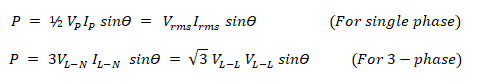# Power Formulas in DC and AC Single-Phase & Three-Phase Circuits

## Electric Power Formulas & Equations in DC and AC 1-Φ & 3-Φ Circuits

Back to basic, below are the simple Electric Power formulas for Single Phase AC Circuit, Three Phase AC Circuits and DC Circuits. You can easily find electric power in watts by using the following electric power formulas in electric circuits.• P = V x I
• P = I2 x R
• P = V2 / R

### Power Formulas in Single Phase AC Circuits

• P = V x I x Cos Ф
• P = I2 x R x Cos Ф
• P = V2 / R (Cos Ф)

### Power Formulas in Three Phase AC Circuits

• P = √3 x VL x IL x Cos Ф
• P = 3 x VPh x IPh x Cos Ф
• P = 3 x I2 x R x Cos Ф
• P = 3 (V2 / R) x Cos Ф

Where:### AC Power Formulas in Complex Circuits:

#### Complex Power & Apparent Power:

When there is an inductor or capacitor in a circuit, the power becomes complex power “S”, meaning it has two parts i.e. real & imaginary part. The magnitude of Complex power is called Apparent power |S|.

• P is the real power
• Q is the reactive power

#### Active or Real Power & Reactive Power:

The real part is Complex power “S” is known as active or real power “P” & the imaginary part is known as reactive power “Q”.

• S = P + jQ
• P = V I cosθ
• Q = V I sinθ

Where

θ is the phase angle between voltage & current.

#### Power Factor:

Power factor “PF” is the ratio of real power “P” to apparent power “|S|”. Mathematically, Power factor is the cosine of angle θ between real power and apparent power.

|S| = √(P2+Q2)

Other formulas used for Power Factor are as follow:

Cosθ = R/Z

Where:

• Cosθ = Power Factor
• R = Resistance
• Z = Impedance (Resistance in AC circuits i.e. XLXC and R known as Inductive reactancecapacitive reactance and resistance respectively).

Cosθ = kW / kVA

Where

• Cosθ = Power Factor
• kW = Real Power in Watts
• kVA = Apparent Power in Volt-Amperes or Watts

Additional formulas used for power factor.

• Cosθ = P / V I
• Cosθ = kW / kVA

#### Real Power Of Single Phase & 3-Phase CurrentWhere

• Vrms  & Irms is the root mean square value of voltage & current respectively.
• VL-N  & IL-N  is the line-to-neutral voltage & current respectively.
•  VL-L & IL-L  is the line-to-line voltage & current respectively.
• Cosθ is the power factor PF.

#### Reactive Power Of Single & 3-Phase Current:Where

θ = is the phase angle i.e. phase difference between voltage and current.

The following table shows the different electrical power formulas in both AC and DC circuits.

 Quantity DC AC (1-Phase) AC (3-Phase) Power (W) P = V x I P = I2 x R P = V2 / R P = V x I x Cos Ф P = I2 x R x Cos Ф P = V2 / R (Cos Ф) P = √3 x VL x IL x Cos Ф P = 3 x VPh x IPh x Cos Ф P = 3 x I2 x R x Cos Ф P = 3 (V2 / R) x Cos Ф

Related Formulas and Equations Posts:

### Electrical Technology

1. Carter Abraham says:

When there is no retreat, the potential power will be exerted.

2. Arsalan Abbas says:

Dear i want to know about, why we rated prim-mover in MW (Mega-Watt) while our Generator rated in KVA………please tell me.

1. MOHAMED IQBAL MOOSA says:

The out put of the Generator will change depending on the load power factor ,0.8, 0.9 or unity power factor which is only in lighting load also if capacitance load is their the KW OR MEGA WATT WILL BE MORE.

This Answer KVA*.8=KW And KW/1000= Mega Watt

3. Divyesh gohil says:

In 45 kw ac drive, output motor consumes 30kw, then another how much dc current use in dc bus.
Means what is the formula od dc current.

4. Aman Kumar says:

Sir i want to know about single phase circuit. pls help me.

1. If you are talking about single phase formulas and MCQS, check it here.

5. M Qasim says:

Dear sir,

6. Mohammed Shiyas says:

7. Naeem Shaukat says:

Sir i want to know in detail about KVA

8. Sunil says:

Formula P=(I^2)*(R)*Cos(A) is P=(I^2)*R.

P=3*(I^2)*(R)*Cos(A) is P=3*(I^2)*R.